﻿ 自适应爆炸半径和差分变异的增强烟花算法 Enhanced Fireworks Algorithm with Adaptive Explosion Amplitude and Differential Variation

Vol.07 No.02(2018), Article ID:23753,11 pages
10.12677/AAM.2018.72019

Enhanced Fireworks Algorithm with Adaptive Explosion Amplitude and Differential Variation

Wenwen Ye, Jiechang Wen

School of Applied Mathematics, Guangdong University of Technology, Guangzhou Guangdong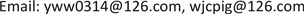Received: Jan. 18th, 2018; accepted: Feb. 3rd, 2018; published: Feb. 13th, 2018ABSTRACT

Enhanced fireworks algorithm improves the performance defect of the fireworks algorithm, and shows good effect in many optimization problems. However, it still has low precision and is easy to fall into local optimal solution prematurely in some functions. In order to improve the above mentioned problems, the maximum explosion amplitude is set as the simulated annealing factor to accelerate its search process, and we learn from the idea of differential variation to increase the diversity of the population, so as to jump out of the local optimal solution. The proposed algorithm is tested on 10 benchmark functions and the experimental results show that the proposed algorithm significantly outperforms the enhanced fireworks algorithm and the fireworks algorithm.

Keywords:Enhanced Fireworks Algorithm, Simulated Annealing, Adaptive Explosion Amplitude, Differential Variation1. 引言

2. 增强烟花算法

$\mathrm{min}f\left(x\right)\in R,x\in \Omega$ (1)

2.1. 爆炸算子

${S}_{i}=m\frac{{y}_{\mathrm{max}}-f\left({x}_{i}\right)+\epsilon }{\underset{i=1}{\overset{N}{\sum }}\left({y}_{\mathrm{max}}-f\left({x}_{i}\right)\right)+\epsilon }$ (2)

${A}_{i}=A\frac{f\left({x}_{i}\right)-{y}_{\mathrm{min}}+\epsilon }{\underset{i=1}{\overset{N}{\sum }}\left(f\left({x}_{i}\right)-{y}_{\mathrm{min}}\right)+\epsilon }$ (3)

${y}_{\mathrm{max}}=\mathrm{max}\left(f\left({x}_{i}\right)\right),\left(i=1,2,\cdot \cdot \cdot ,N\right),\text{\hspace{0.17em}}\text{\hspace{0.17em}}{y}_{\mathrm{min}}=\mathrm{min}\left(f\left({x}_{i}\right)\right),\left(i=1,2,\cdot \cdot \cdot ,N\right)$

${\stackrel{^}{s}}_{i}=\left\{\begin{array}{l}round\left(am\right),Sbm,a (4)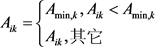(5)

${A}_{\mathrm{min},k}\left(t\right)={A}_{init}-\frac{{A}_{init}-{A}_{final}}{eval{s}_{\mathrm{max}}}\sqrt{\left(2eval{s}_{\mathrm{max}}-t\right)t}$ (6)

2.2. 变异操作

${x}_{ik}={x}_{ik}+\left({x}_{Bk}-{x}_{ik}\right)×e$ (7)

2.3. 映射规则

EFWA为了避免原始烟花算法造成智能加速收敛的假象，提出一种随机映射规则，其公式为：

${x}_{ik}={x}_{lb,k}+U\left(0,1\right)\cdot \left({x}_{ub,k}-{x}_{lb,k}\right)$ (8)

2.4. 选择策略

3. 自适应爆炸半径和差分变异的增强烟花算法

SAEFA是在EFWA的基础上改进的。它们的不同之处是改进的算法将最大爆炸半径设置为模拟退火因子，并且借鉴了差分变异的思想将新型高斯变异方式变为差分变异方式。改进的算法由爆炸算子、变异操作和选择操作组成，同时为了防止越界也使用了映射规则。

3.1. 最大爆炸半径的设置

EFWA中将最大爆炸半径设置为常数，这将造成算法后期精细搜索能力被减弱，使算法的求解精度不高。受到动态搜索思想的启发，若最大爆炸半径总体上呈现出非线性递减的变化趋势，算法前期将有利于全局搜索，后期则有利于局部搜索，达到自适应加速的效果。

Step1：初始温度 $T$ ，初始解状态 $S$ ，每个 $T$ 值的迭代次数 $L$

Step2：对 $k=1,\cdot \cdot \cdot ,L$ 执行Step3至Step6；

Step3：产生新解 ${S}^{\prime }$

Step4：计算增量 $\Delta {t}^{\prime }=C\left({S}^{\prime }\right)-C\left(S\right)$ ，其中 $C\left(S\right)$ 为评价函数；

Step5：若 $\Delta {t}^{\prime }<0$ ，则新解为 ${S}^{\prime }$ ，否则以概率 $\mathrm{exp}\left(-\Delta {t}^{\prime }/kT\right)$ 接受 ${S}^{\prime }$ 为新的当前解；

Step6：终止条件满足则输出 ${S}^{\prime }$ 作为最优解；

Step7： $T$ 逐渐减少趋于0，转至Step2。

$R$ 为初始最大爆炸半径， $Gen$ 为当前迭代次数， $MaxGen$ 为最高的迭代次数，则第 $i$ 个烟花最大爆炸半径公式为：

${R}_{i}=R{\left(1-\frac{Gen}{MaxGen}\right)}^{5}$ (9)

${A}_{i}={R}_{i}\frac{f\left({x}_{i}\right)-{y}_{\mathrm{min}}+\epsilon }{\underset{i=1}{\overset{N}{\sum }}\left(f\left({x}_{i}\right)-{y}_{\mathrm{min}}\right)+\epsilon }$ (10)

3.2. 变异操作

${x}_{i}^{k}={c}_{1}{x}_{best}^{k}+{c}_{2}\left({x}_{j1}^{k}-{x}_{j2}^{k}\right)$ (11)

3.3. 基于模拟退火和差分变异的搜索策略

SAEFA沿用了EFWA计算爆炸火花的数量公式，采用同样的爆炸火花产生方式和选择策略，而采用公式(10)计算爆炸半径和采用公式(11)进行变异操作。SAEFA的基本流程如下：

Step1：初始时随机生成 $n$ 个烟花的位置；

Step2：计算所有烟花的适应度值；

Step3：循环执行a至e，直到满足终止条件；

a：使用公式(2)和(4)计算烟花个数；

b：使用公式(10)计算烟花的爆炸半径；

c：生成爆炸火花；

d：使用公式(11)生成变异火花；

e：用选择策略选择 $N$ 个个体进入下一代；

Step4：输出最优个体的位置和其适应度值。

4. 实验设置和结果分析

4.1. 实验设置

4.2. 实验结果分析Table 3. Comparison of global optimal mean of Sphere function (SI is offset index)Table 4. Comparison of global optimal mean of Schwefel function (SI is offset index)Table 5. Comparison of global optimal mean of Ackley function (SI is offset index)Table 6. Comparison of global optimal mean of Griewank function (SI is offset index)Table 7. Comparison of global optimal mean of Penalized function (SI is offset index)Table 8. Comparison of global optimal mean of F 1 ( x ) function (SI is offset index)Table 9. Comparison of global optimal mean of Goldstein-Price function (SI is offset index)Table 10. Comparison of global optimal mean of Schaffer function (SI is offset index)Table 11. Comparison of global optimal mean of F 2 ( x ) function (SI is offset index)Table 12. Comparison of global optimal mean of F 3 ( x ) function (SI is offset index)

5. 总结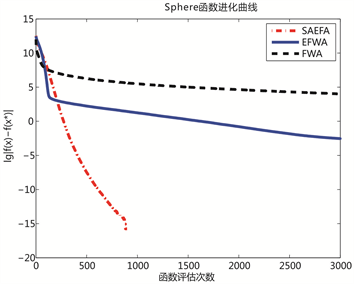Figure 1. Convergence curves of Sphere and Schwefel functions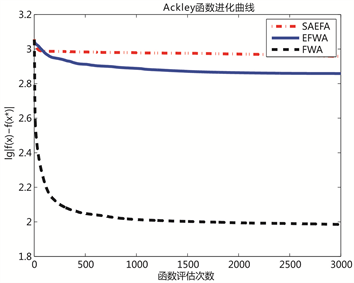Figure 2. Convergence curves of Ackley and Generalize-Griewank functions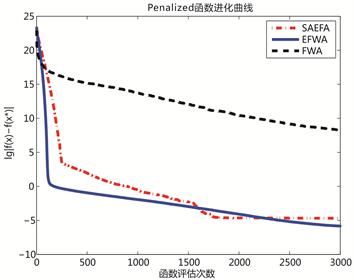Figure 3. Convergence curves of Penalized and ${F}_{1}\left(x\right)$ functions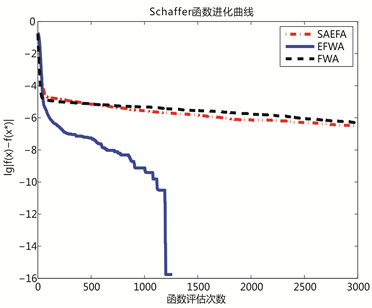Figure 4. Convergence curves of Goldstein and Schaffer functions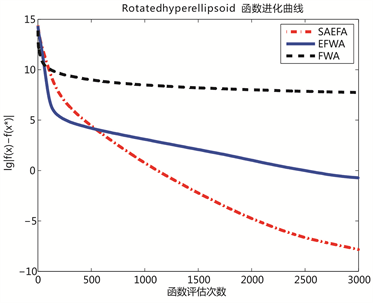Figure 5. Convergence curves of ${F}_{2}\left(x\right)$ and ${F}_{3}\left(x\right)$ functions

Enhanced Fireworks Algorithm with Adaptive Explosion Amplitude and Differential Variation[J]. 应用数学进展, 2018, 07(02): 152-162. http://dx.doi.org/10.12677/AAM.2018.72019

1. 1. Tan, Y. and Zhu, Y. (2010) Fireworks Algorithm for Optimization. International Conference on Advances in Swarm Intelligence. Springer-Verlag, 355-364.

2. 2. Pei, Y., Zheng, S., Tan, Y. and Hideyuki, T. (2012) An Empirical Study on Influence of Approximation Approaches on Enhancing Fireworks Algorithm. 2014 IEEE International Conference on Systems, 1322-1327.
https://doi.org/10.1109/ICSMC.2012.6377916

3. 3. Si, T. and Ghosh, R. (2015) Explosion Sparks Generation Using Adaptive Transfer Function in Firework Algorithm. 2015 IEEE International Conference on Signal Processing, Communication and Networking, 1-9.
https://doi.org/10.1109/ICSCN.2015.7219917

4. 4. Zheng, S., Janecek, A. and Tan, Y. (2013) Enhanced Fire-works Algorithm. 2013 IEEE Congress on Evolutionary Computation (CEC), 2069-2077.
https://doi.org/10.1109/CEC.2013.6557813

5. 5. Zheng, S.Q., Janecek, A., Li, J.Z. and Tan, Y. (2014) Dynamic Search in Fireworks Algorithm. 2014 IEEE Congress on Evolutionary Computation (CEC), 3222-3229.
https://doi.org/10.1109/CEC.2014.6900485

6. 6. Li, J., Zheng, S. and Tan, Y. (2014) Adaptive Fireworks Algo-rithm. 2014 IEEE Congress on Evolutionary Computation (CEC), 3214-3221.
https://doi.org/10.1109/CEC.2014.6900418

7. 7. Yu, C., Li, J. and Tan, Y. (2014) Improve Enhanced Fireworks Algorithm with Differential Mutation. IEEE International Conference on Systems, Man and Cybernetics. IEEE, 264-269.

8. 8. Zheng, Y.J., Xu, X.L., Ling, H.F. and Chen, S.Y. (2015) A Hybrid Fireworks Optimization Method with Differential Evolution Operators. 3rd International Conference on Swarm Intelligence (ICSI), 75-82

9. 9. Zheng, S.Q., Li, J.Z., Janecek, A. and Tan, Y. (2016) A Cooperative Framework for Fireworks Algorithm. IEEE/ACM Transactions on Computational Biology and Bioinformatics, 1-14.

10. 10. Zhang, B., Zheng, Y.J., Zhang, M.X., et al. (2017) Fireworks Algorithm with Enhanced Fireworks Interaction. IEEE/ACM Transactions on Computational Biology & Bioinformatics, 14, 42.
https://doi.org/10.1109/TCBB.2015.2446487

11. 11. Li, J., Zheng, S. and Tan, Y. (2017) The Effect of Information Utilization: Introducing a Novel Guiding Spark in the Fireworks Algorithm. IEEE Transactions on Evolutionary Computation, 21, 153-166.
https://doi.org/10.1109/TEVC.2016.2589821

12. 12. Gao, H. and Diao, M. (2011) Cultural Firework Algorithm and Its Application for Digital Filters Design. International Journal of Modelling Identification & Control, 14, 324-331.
https://doi.org/10.1504/IJMIC.2011.043157

13. 13. 赵晶, 唐焕文, 朱训芝. 模拟退火算法的一种改进及其应用研究[J]. 大连理工大学学报, 2006, 46(5): 775-780.

14. 14. Storn, R. and Price, K. (1997) Differential Evolution—A Simple and Efficient Heuristic for Global Optimization over Continuous Spaces. Journal of Global Optimization, 11, 341-359.
https://doi.org/10.1023/A:1008202821328

15. 15. Liang, J., Nsuganthan, P. and Ghernandez Diaz, A. (2013) Problem Definitions and Evaluation Criteria for the CEC2013 Special Session on Real-Parameter Opti-mization.

16. 16. Liang, J.J., Qu, B.Y. and Nsuganthan, P. (2014) Problem Definitions and Evaluation Criteria for the CEC2013 Special Session and Competition on Single Objective Real-Parameter Numerical Optimization.